Learn your way!

## Writing T-SQL Queries in SQL Server 2008

#### Course at a glance

Included in these subscriptions:

• Dev & IT Pro Video
• Dev & IT Pro Power Pack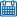Release date 2/25/2009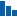Level Advanced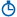Runtime 21h 49m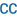Closed captioning N/A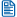Transcript N/A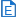eBooks / courseware Included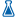Hands-on labs Included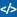Sample code Included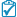Exams Included##### Enterprise Solutions

Need reporting, custom learning tracks, or SCORM? Learn More

#### Course description

In this course, you will learn how to build basic queries using Transact-SQL, the language of SQL Server. Then, you will learn how to build effective views, stored procedures, triggers, and user-defined functions, using Transact-SQL. You will learn about the new enhancements to the Transact-SQL programming language including improved support for error handling and hierarchical queries.

#### Meet the expertDon Kiely is a featured instructor on many of our SQL Server and Visual Studio courses. He is a nationally recognized author, instructor, and consultant specializing in Microsoft technologies. Don has many years of teaching experience, is the author or co-author of several programming books, and has spoken at many industry conferences and user groups. In addition, Don is a consultant for a variety of companies that develop distributed applications for public and private organizations.

#### Module 1

##### Tour of SQL 08 (34:46)
• Introduction (03:11)
• Editions (03:29)
• Server Components (02:44)
• Management Tools (02:38)
• Documentation (00:52)
• Feature Pack (01:24)
• SSMS (00:49)
• Demo: SSMS (19:11)
• Summary (00:24)
##### Server Activity (36:56)
• Introduction (00:50)
• Demo: Activity Monitor (14:27)
• Tables, Queries, Views (00:28)
• Demo: Table Designer (03:15)
• Demo: Template Explorer (08:25)
• SSIS (03:03)
• BIDS (00:54)
• Demo: BIDS (02:09)
• Reporting Services (01:44)
• SSAS (01:07)
• Summary (00:30)

#### Module 2

##### Relational Design (37:44)
• Introduction (01:34)
• Design Principles (00:44)
• Key Terms (02:32)
• Relational Theory (01:07)
• Surrogate Keys (01:50)
• Demo: DB Design (01:32)
• Data Normalization (00:32)
• First Normal Form (03:17)
• Second Normal Form (02:58)
• Third Normal Form (01:42)
• Computed Columns (00:43)
• Key Dependence (00:49)
• Postal Lookup Tables (00:40)
• Beyond Normal Forms (00:54)
• Referential Integrity (01:40)
• Relationships (03:12)
• One-to-One (03:08)
• Enforcing Integrity (00:58)
• Cascading (00:52)
• Cascading Updates (00:41)
• Cascading Deletes (00:55)
• Cascading Actions (01:24)
• Beyond Normalization (02:01)
• Business Rules (01:19)
• Summary (00:28)
##### Implementation (17:48)
• Introduction (00:43)
• Database Storage (01:19)
• Storage (02:47)
• Data Integrity (01:40)
• Recovery Models (01:28)
• Demo: Implementation (04:51)
• Rules for Identifiers (01:50)
• Demo: DB Properties (02:03)
• Summary (01:03)

#### Module 3

##### Data Types (01:02:41)
• Introduction (00:55)
• Character-based (03:13)
• Numeric Data (04:18)
• Date and Time (05:11)
• Binary Types (01:37)
• Identifier Types (03:11)
• sql_variant (00:56)
• Variable-only (01:49)
• XML Type (01:42)
• System SQLCLR Types (02:26)
• Demo: Data Types (10:17)
• Creating Constraints (03:50)
• Demo: Constraints (08:12)
• Triggers (03:15)
• Creating Indexes (00:33)
• Demo: Index (10:31)
• Summary (00:37)

#### Module 4

##### Management Studio (31:18)
• Introduction (01:23)
• Demo: Connecting (03:25)
• Configure Options (08:16)
• Customize Toolbars (00:35)
• Menu Items (03:46)
• Query Designer (00:55)
• Projects and Solutions (04:17)
• Object Explorer (02:06)
• Filter Nodes (02:15)
• View Dependencies (01:10)
• Object Details (01:21)
• Designers (01:11)
• Summary (00:32)
##### Query Editor (44:42)
• Introduction (00:53)
• Demo: Overview (03:06)
• Editing Options (07:07)
• Script Table as (01:18)
• Stored Procedure (02:19)
• Debugging (07:07)
• Template Explorer (03:27)
• Execution Plans (04:08)
• Results to a File (01:06)
• SQLCMD Mode (01:04)
• New Project (04:59)
• Working Offline (03:37)
• Books Online (00:36)
• Demo: Help Features (03:19)
• Summary (00:30)

#### Module 5

##### Transact SQL (31:37)
• Introduction (00:32)
• T-SQL Extensions (02:40)
• Batches and Scripts (00:52)
• Demo: Batches (10:45)
• Data Type Precedence (01:08)
• Demo: Converting Types (06:00)
• Built-In Functions (01:41)
• Working with Nulls (01:34)
• Demo: Nulls (05:50)
• Summary (00:30)
##### Handling Functions (35:29)
• Introduction (00:52)
• Demo: ISNUMERIC (02:05)
• Using RAND (02:02)
• ROUND (03:13)
• REPLACE (01:46)
• STUFF (00:53)
• LEN, LEFT, RIGHT (01:19)
• SUBSTRING (00:54)
• CHARINDEX (02:22)
• PATINDEX (02:54)
• SPACE (01:01)
• CHAR and ASCII (00:51)
• LOWER and UPPER (03:17)
• LTRIM and RTRIM (00:53)
• GETDATE (00:17)
• MONTH, DATE, YEAR (00:12)
• DATEPART (01:02)
• DATENAME (00:29)
• DATE Math (02:19)
• Global Functions (06:05)
• Summary (00:32)

#### Module 6

##### Controlling Flow (23:11)
• Introduction (00:49)
• Demo: IF...ELSE (01:14)
• BEGIN...END (01:30)
• GOTO (02:02)
• RETURN (01:37)
• CASE (06:13)
• WHILE (01:49)
• WAITFOR (01:49)
• Ranking Results (01:22)
• Demo: Ranking (04:14)
• Summary (00:26)
##### Transactions (33:04)
• Introduction (01:25)
• Passing the ACID Test (02:25)
• Transaction Types (01:56)
• Transaction Details (01:01)
• Isolation Levels (01:28)
• Locking (02:11)
• Viewing Lock Info (01:11)
• Avoiding Blocks (00:39)
• Demo: Locking (03:48)
• Deadlocks (03:32)
• Preventing (02:48)
• Applications (00:32)
• Designing (01:32)
• Efficient Transactions (02:01)
• Compile\Runtime Errors (01:15)
• Demo: Errors (04:33)
• Summary (00:41)
##### Explicit Transactions (18:00)
• Introduction (00:52)
• Syntax (02:16)
• Demo: Explicit (01:56)
• Error Handling (01:11)
• @@ERROR (00:50)
• Demo: @@ERROR (02:14)
• Stored Procedures (02:05)
• Demo: Stored Procedure (05:58)
• Summary (00:34)

#### Module 7

##### Raise Error Try/Catch (18:24)
• Introduction (01:10)
• Demo: RAISERROR (00:42)
• User-Defined Errors (02:08)
• TRY/CATCH Overview (00:39)
• Demo: TRY/CATCH (01:00)
• Error Chaining (01:07)
• Errors Not Handled (02:17)
• Demo: Error Info (05:15)
• XACT_STATE (02:04)
• Demo: XACT_STATE (01:16)
• Summary (00:42)
##### Data Selection Queries (36:00)
• Introduction (01:35)
• Transact-SQL (02:14)
• Schemas and Naming (03:34)
• Demo: T-SQL Code (18:08)
• Three-Valued Logic (02:31)
• Demo: Nulls (07:31)
• Summary (00:25)
##### Sorting (43:53)
• Introduction (01:13)
• Demo: Order By (02:11)
• Group By Clause (00:57)
• Aggregate Functions (01:14)
• Demo: Count Rows (07:42)
• Demo: Top (02:13)
• Joining Tables (01:09)
• Demo: Join Tables (13:39)
• Demo: Outer Join (05:54)
• Demo: Inner & Outer (07:09)
• Summary (00:27)

#### Module 8

##### HierarchyID (55:12)
• Introduction (02:14)
• Overview (02:33)
• Indexing a Hierarchy (00:42)
• Depth-first (01:27)
• Breadth-first (01:24)
• Manipulating (01:41)
• Demo: HierarchyID (16:22)
• Sparse Columns (02:08)
• Restrictions (02:15)
• Column Sets (01:01)
• Restrictions (00:45)
• Recommendations (00:37)
• Demo: Sparse Columns (06:02)
• Column Sets (06:27)
• Filtered Indexes (02:15)
• Using Filtered Indexes (01:48)
• Demo: Filtered Indexes (04:57)
• Summary (00:25)

#### Module 9

##### FileStream Spatial Data (52:39)
• Introduction (02:53)
• FILESTREAM Storage (01:18)
• Data Access Methods (01:17)
• Gotchas & Limitations (01:39)
• Demo: FILESTREAM (09:41)
• Spatial Data (01:04)
• Geometry vs. Geography (03:28)
• Geometric Plane (00:46)
• Mother Ship (00:28)
• Projection Distortion (02:41)
• Data Standards (01:33)
• Types of Spatial Data (01:35)
• Spatial Objects (01:54)
• Geospatial Data (02:03)
• Demo: Spatial Data (19:36)
• Summary (00:35)
##### Complex Queries (25:43)
• Introduction (00:35)
• NULL Values (00:21)
• SQLTypes and CLR Types (04:25)
• Demo: Uninitialized Variables (04:02)
• ANSI_NULLS Option (02:40)
• Demo: ANSI_NULLS (02:09)
• NULLS and SQLBoolean (01:39)
• Demo: SQLBoolean (02:53)
• Assigning NULL Values (00:32)
• CLR Integration (01:16)
• Direct Assignment (00:56)
• NULLIF (00:15)
• COALESCE (00:41)
• Demo: Assigning NULLS (02:51)
• Summary (00:21)
##### Ranking (16:35)
• Introduction (00:34)
• Ranking Grouped Data (01:02)
• ROW_NUMBER (02:38)
• RANK (01:42)
• DENSE_RANK (01:09)
• NTILE (04:01)
• Demo: ROW_NUMBER (01:42)
• RANK (01:15)
• DENSE_RANK (00:53)
• NTILE (01:21)
• Summary (00:15)

#### Module 10

##### Correlated Subqueries (17:20)
• Introduction (00:34)
• Writing Subqueries (01:08)
• Subquery Basics (01:15)
• Correlated Subquery (00:36)
• Demo: Subquery (01:14)
• Correlated (01:53)
• WHERE Clause (01:55)
• ANY, SOME, ALL (00:45)
• HAVING Clause (00:23)
• Updates (00:38)
• Demo: UPDATE (01:28)
• Joins and Temp Tables (02:59)
• Demo: Using a Join (02:04)
• Summary (00:20)
##### Common Table Expressions (16:32)
• Introduction (00:42)
• When to Use CTEs (01:46)
• CTE Syntax (00:50)
• Overview (00:01)
• Demo: Defining a CTE (02:49)
• Recursive CTEs (04:33)
• Demo: Recursive CTE (05:30)
• Summary (00:17)
##### Modifying Data (35:15)
• Introduction (01:17)
• Inserting Data (00:27)
• Demo: INSERT (19:14)
• Temporary Tables (01:44)
• Demo: Temp Tables (02:31)
• Uses for Temp Tables (02:24)
• Demo: Global Temps (07:03)
• Summary (00:33)

#### Module 11

##### Updating Data (32:50)
• Introduction (00:42)
• Demo: Update Statement (08:05)
• Demo: With Transaction (02:12)
• Demo: Deleting Data (03:28)
• Transaction Isolation (02:20)
• Isolation Levels (04:12)
• Blocking and Deadlocks (01:46)
• Deadlocks (03:08)
• Demo: Isolation Levels (02:11)
• Snapshot Isolation (01:54)
• Caveats (00:38)
• Demo: Snapshot Isolation (01:42)
• Summary (00:26)
##### XML Data Type (21:16)
• Introduction (00:42)
• Overview (01:01)
• Declaring XML Objects (05:01)
• Loading Data (00:39)
• SELECT...FOR XML (01:40)
• OPENROWSET (01:43)
• Demo: Direct Assign (04:30)
• Indexing Columns (00:45)
• Primary Indexes (01:18)
• Secondary Indexes (01:43)
• Demo: Indexes (01:44)
• Summary (00:23)

#### Module 12

##### XML Schema Collections (22:08)
• Introduction (00:52)
• Overview (01:07)
• Typed and Untyped XML (01:35)
• Schema Basics (01:18)
• Demo: XSD (02:34)
• Lax Validation (03:12)
• Full DateTime Support (01:02)
• Registering Schemas (01:54)
• Viewing Stored Schemas (01:46)
• Collections (00:29)
• Namespaces (00:18)
• Components (00:45)
• Namespace (01:30)
• Demo: Schema Info (03:15)
• Summary (00:23)
##### Querying XML (25:17)
• Introduction (00:41)
• Overview (00:44)
• Using XQuery (01:02)
• query() (00:30)
• value() (00:48)
• exist() (00:36)
• modify() (00:25)
• nodes() (00:36)
• Using XQuery (00:34)
• Demo: XQuery Methods (04:03)
• FLWOR (03:49)
• FOR XML (01:05)
• Demo: FOR XML (04:21)
• OPENXML (02:26)
• Demo: OPENXML (03:04)
• Summary (00:26)
##### Best Practices (09:11)
• Introduction (00:40)
• Overview (00:48)
• Relational vs. XML (02:03)
• Storing XML (01:19)
• Reasons to Index (02:22)
• Querying (01:24)
• Summary (00:32)

#### Module 13

##### Views (36:22)
• Introduction (01:42)
• Advantages of Views (01:17)
• Views and Security (01:04)
• Creating Views (00:57)
• View Rules (01:40)
• Views and ORDER BY (02:05)
• Syntax and Options (01:21)
• CHECK OPTION (01:02)
• Other Options (01:46)
• Tools for Creating Views (00:36)
• Demo: Creating Views (05:10)
• From Templates (01:30)
• Edit Views (00:52)
• View Examples (08:23)
• Nesting Views (02:25)
• CTE (01:48)
• Encrypting (02:06)
• Summary (00:30)
##### Updating View Data (24:36)
• Introduction (00:51)
• Updating Rules (00:54)
• Updating Behavior (00:55)
• Demo: Limiting Updates (05:18)
• Using Computed Columns (02:20)
• Demo: Computed Columns (02:16)
• Indexed Views (01:27)
• How Indexed View Work (01:03)
• Performance Benefits (01:27)
• Data Modifications (01:00)
• Requirements (01:51)
• Demo: Indexed Views (02:16)
• Partitioned Views (02:10)
• Summary (00:41)

#### Module 14

##### SQL Server Partitions (20:54)
• Introduction (00:44)
• Overview (00:50)
• Why Partition? (01:55)
• Partitioning (00:44)
• 6.5 and Earlier (01:09)
• SQL Server 7.0 (01:31)
• SQL Server 2000 (00:47)
• SQL Server 2005/2008 (00:52)
• SQL Server 2008 (01:04)
• Range Partitions (01:28)
• Partition Key (02:25)
• Multiple Filegroups (04:49)
• Index Partitioning (02:05)
• Summary (00:24)
##### Creating Partitioned Tables (33:09)
• Introduction (00:38)
• Overview (02:37)
• LEFT and RIGHT (04:52)
• Datetime Values (02:09)
• RANGE RIGHT (01:04)
• Demo: Partition Function (01:39)
• Partition Scheme (06:24)
• Demo: Filegroups (02:02)
• Partition Scheme (01:39)
• Tables and Indexes (03:16)
• Demo: Partitioned Table (02:41)
• Partitioned Index (03:35)
• Summary (00:27)
##### Querying Partitions (18:23)
• Introduction (00:30)
• Overview (01:29)
• \$PARTITION Function (02:00)
• Demo: \$PARTITION (05:59)
• Catalog Views (02:05)
• sys.partition_functions (00:53)
• sys.partition_range_values (00:22)
• sys.partition_schemes (00:37)
• sys.data_spaces (00:31)
• Demo: Catalog Views (03:28)
• Summary (00:23)

#### Module 15

##### Managing Partitions (21:54)
• Introduction (00:35)
• Overview (00:43)
• Tables or Indexes (00:51)
• Partition Function (01:53)
• Splitting Partitions (02:12)
• Merging Partitions (02:20)
• Switching Partitions (03:36)
• Partition Scheme (01:45)
• Backing Up Partitions (02:20)
• Performance (00:36)
• Join Queries (00:56)
• Multiple Disk Drives (00:58)
• Lock Escalation (02:34)
• Summary (00:27)
##### User Defined Functions (18:43)
• Introduction (02:28)
• Scalar Functions (01:39)
• WITH Function Options (02:05)
• Demo: Scalar Functions (02:37)
• With Parameters (02:04)
• Inline Functions (00:51)
• Inline Syntax (00:28)
• Demo: Inline Function (02:11)
• Inline Parameters (01:35)
• Updating Data (02:03)
• Summary (00:36)
##### Table Valued Functions (17:54)
• Introduction (00:52)
• Multi-Statement Syntax (00:12)
• Limitations (01:25)
• TABLE Data Type (01:03)
• Demo: Multi-Statement (06:38)
• Using Functions, Views... (00:42)
• Demo: Scalar Functions (02:05)
• Table-Valued Functions (02:16)
• Choosing Between (02:04)
• Summary (00:33)

#### Module 16

##### Stored Procedures (36:39)
• Introduction (01:00)
• Overview (03:15)
• Performance Benefits (01:50)
• Deferred Name Resolution (01:04)
• Execution Plans (01:54)
• Plan Recompilation (01:45)
• Using Schema Qualifiers (01:37)
• Reasons to Use (02:08)
• Stored Procedure Syntax (01:31)
• Creating Procedures (02:35)
• SET NOCOUNT ON (02:12)
• Parameters (02:06)
• Optional Parameters (02:02)
• Output Parameters (02:42)
• Return (03:25)
• Variables (03:34)
• Debugging (01:28)
• Summary (00:22)
##### Testing and Triggers (28:06)
• Introduction (01:36)
• Demo: Debugging (03:43)
• PRINT Statements (02:20)
• Output Parameters (03:04)
• Creating Triggers (01:42)
• Types of Triggers (01:37)
• Uses for Triggers (01:05)
• Trouble with Triggers (02:02)
• How Triggers Work (01:42)
• Demo: After Trigger (03:05)
• INSTEAD OF Trigger (03:20)
• DDL Trigger (02:14)
• Summary (00:30)
##### Full-Text Search (29:27)
• Introduction (02:08)
• Demo: Full-Text Index (06:58)
• Full-Text Queries (01:03)
• CONTAINS Predicate (02:12)
• Demo: Simple Terms (01:06)
• Generation Terms (06:21)
• Prefix Terms (00:59)
• Proximity Terms (00:31)
• FREETEXT (01:19)
• CONTAINSTABLE (02:26)
• Ranking Relevance (03:48)
• Summary (00:30)

#### Module 17

##### Advanced TSQL (54:33)
• Introduction (00:58)
• Using APPLY (00:49)
• Demo: APPLY With Joins (06:33)
• APPLY With TVF (02:53)
• FOR XML PATH (01:30)
• MERGE (02:32)
• Five Clauses in MERGE (00:49)
• WHEN Clause (03:02)
• Demo: MERGE (06:22)
• Recursive Queries (01:13)
• Demo: CTE (05:35)
• Grouping Sets (00:52)
• Demo: Base Query (02:35)
• Grouping Sets (06:11)
• ROLLUP (02:13)
• CUBE (03:12)
• GROUPING_ID (02:36)
• Pivot Queries (00:49)
• Demo: Pivot (03:08)
• Summary (00:32)
##### Executing Dynamic SQL (25:31)
• Introduction (00:52)
• Demo: Dynamic SQL (03:32)
• sp_executesql (00:33)
• Demo: Parameters (04:26)
• QUOTENAME (05:58)
• Output Parameters (01:19)
• Signing Stored Procedures (03:10)
• Demo: Certificates (05:04)
• Summary (00:34)

#### Module 18

##### Complex Data And Structures (28:54)
• Introduction (00:32)
• Overview (00:19)
• Issues with Data Types (02:50)
• Demo: Explicit Conversion (02:06)
• Multinational Data (05:52)
• Demo: COLLATE (03:00)
• Hierarchical Data (05:46)
• Demo: hierarchyid (07:53)
• Summary (00:33)
##### Efficient Queries (33:40)
• Introduction (00:51)
• Overview (00:56)
• EXISTS Clause (01:15)
• Join vs. Subqueries (02:55)
• One-Pass Queries (00:46)
• Demo: UNION ALL (01:51)
• One-Pass (01:39)
• Multiple Resultsets (00:44)
• Temp Tables (02:38)
• Table Variables (02:29)
• UDFs (02:29)
• Demo: UDFs (06:28)
• CTEs (00:46)
• Worktables (00:40)
• User-Defined Table Types (01:14)
• Demo: Table Types (04:53)
• Summary (00:58)
##### Working With Complex Queries (19:54)
• Introduction (00:54)
• Overview (00:50)
• Implicit Transactions (03:03)
• Demo: Implicit Set On (03:07)
• Keeping it Simple (02:19)
• Demo: Sub Tasks (04:40)
• UDF (01:48)
• Demo: Procedure (02:47)
• Summary (00:21)
##### Maintaining Query Files (24:33)
• Introduction (00:48)
• Overview (01:29)
• Source Control (02:18)
• SourceSafe (02:48)
• Files Under Control (00:50)
• Demo: Source Control (03:52)
• Keyword Expansion (02:24)
• Enabling (01:27)
• Demo: srcsafe.ini (03:21)
• Version Info (00:30)
• File Versions (00:39)
• Demo: Versions (03:31)
• Summary (00:30)